# Rubykaigi2010mrkn bigdecimal

Aug. 28, 2010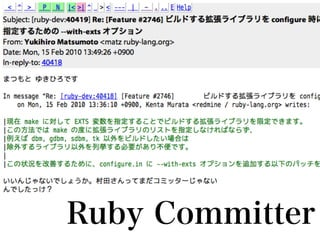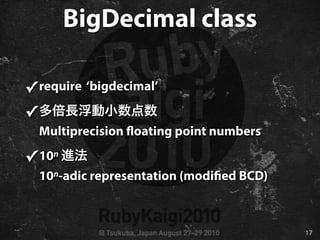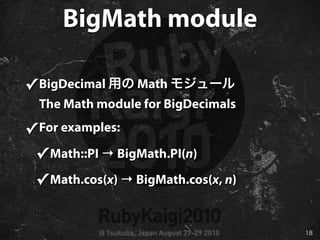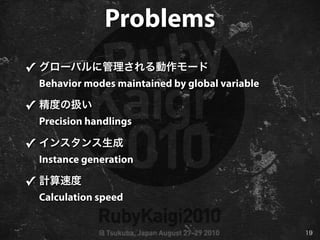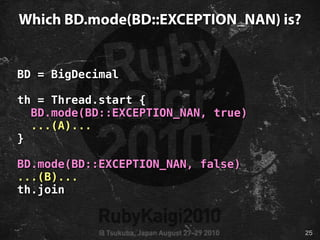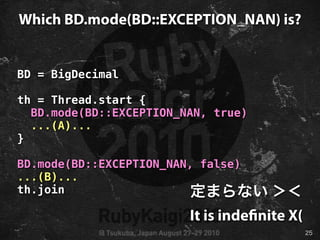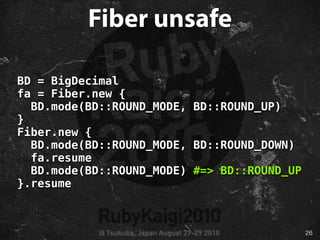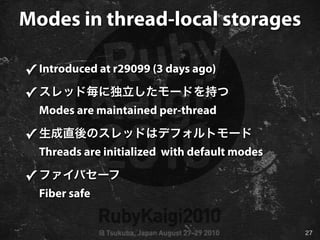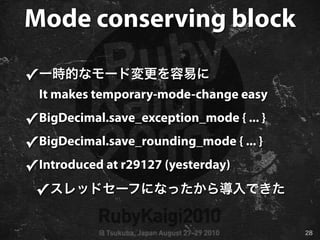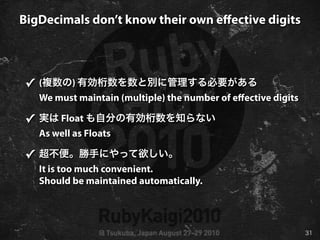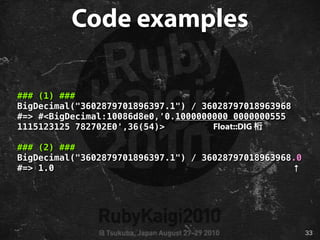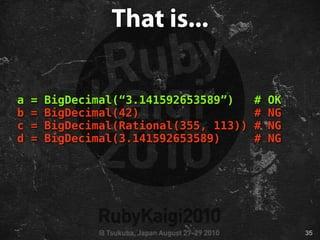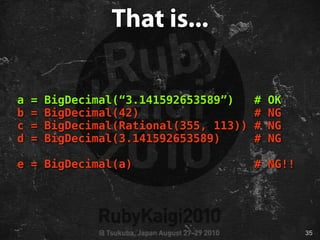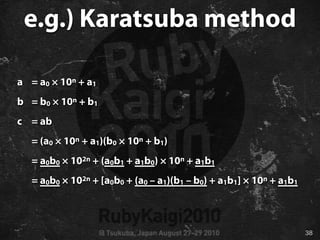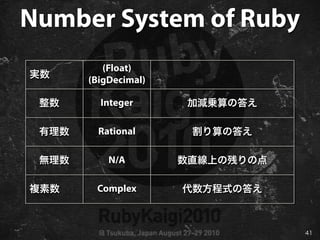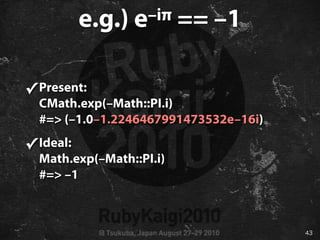1 of 52

### Rubykaigi2010mrkn bigdecimal

• 1. bigdecimal Ruby The future of the bigdecimal library and the number system of Ruby mrkn, Kenta Murata (Genetic Lab Co., Ltd)
• 3. Sapporo is a beautiful provincial city of Japan. Photo by enggul
• 4. from Sapporo, with Love for Ruby Photo by darashi
• 13. Sapporo Kaigi 03 03 MIX 1 3 1 5
• 15. bigdecimal Ruby The future of the bigdecimal library and the number system of Ruby mrkn, Kenta Murata (Genetic Lab Co., Ltd)
• 16. The bigdecimal library ✓ BigDecimal class ✓ BigMath module
• 17. BigDecimal class ✓ require ‘bigdecimal’ ✓ Multiprecision oating point numbers ✓ 10n 10n-adic representation (modi ed BCD)
• 18. BigMath module ✓ BigDecimal Math The Math module for BigDecimals ✓ For examples: ✓ Math::PI → BigMath.PI(n) ✓ Math.cos(x) → BigMath.cos(x, n)
• 19. Problems ✓ Behavior modes maintained by global variable ✓ Precision handlings ✓ Instance generation ✓ Calculation speed
• 20. Modes of BigDecimal ✓ BigDecimal Controlling the behaviors of the system of BigDecimal class ✓ Exception handling mode ✓ ( ) Rounding mode
• 21. Exception handling mode ✓ ✓ In nity ✓ NaN ✓ Under ow ✓ Over ow ✓ Division by zero
• 22. Rounding modes ✓ Round up ✓ Round down (toward zero) ←IEEE754 ✓ Round half up ✓ Round half down ✓ Banker’s rounding ←IEEE754 ✓ Floor (toward +∞) ←IEEE754 ✓ Ceiling (toward –∞) ←IEEE754
• 23. BigDecimal.mode ✓ / The class method for getting or setting modes ✓ Maintained per-process ✓ That is a global variable
• 24. Global modes ✓ Thread unsafe ✓ 2 Cannot simultaneously start two threads which use diﬀerent modes ✓ Fiber unsafe
• 25. Which BD.mode(BD::EXCEPTION_NAN) is? BD = BigDecimal th = Thread.start { BD.mode(BD::EXCEPTION_NAN, true) ...(A)... } BD.mode(BD::EXCEPTION_NAN, false) ...(B)... th.join
• 26. Which BD.mode(BD::EXCEPTION_NAN) is? BD = BigDecimal th = Thread.start { BD.mode(BD::EXCEPTION_NAN, true) ...(A)... } BD.mode(BD::EXCEPTION_NAN, false) ...(B)... th.join It is inde nite X(
• 27. Fiber unsafe BD = BigDecimal fa = Fiber.new { BD.mode(BD::ROUND_MODE, BD::ROUND_UP) } Fiber.new { BD.mode(BD::ROUND_MODE, BD::ROUND_DOWN) fa.resume BD.mode(BD::ROUND_MODE) #=> BD::ROUND_UP }.resume
• 28. Modes in thread-local storages ✓ Introduced at r29099 (3 days ago) ✓ Modes are maintained per-thread ✓ Threads are initialized with default modes ✓ Fiber safe
• 29. Mode conserving block ✓ It makes temporary-mode-change easy ✓ BigDecimal.save_exception_mode { ... } ✓ BigDecimal.save_rounding_mode { ... } ✓ Introduced at r29127 (yesterday) ✓
• 30. Eﬀective digits ✓ The number of eﬀective digits ✓ : 3 (three digits are eﬀective) 3.141592653589792...×100 ↓ 0.314 1592653589792...×101 ✓
• 31. BigDecimal#precs ✓ prec The allocated length of the digit array ✓ prec The used length of the digit array ✓ Not the number of eﬀective digits ✓ Do you use them?
• 32. BigDecimals don’t know their own eﬀective digits ✓ ( ) We must maintain (multiple) the number of eﬀective digits ✓ Float As well as Floats ✓ It is too much convenient. Should be maintained automatically.
• 33. Collaborate with Floats ✓ Float Force converted into Floats ✓ Float::DIG The number of digits is forced to Float::DIG ✓ It is dangerous, don’t mix them!
• 34. Code examples ### (1) ### BigDecimal("3602879701896397.1") / 36028797018963968 #=> #<BigDecimal:10086d8e0,'0.1000000000 0000000555 1115123125 782702E0',36(54)> Float::DIG ### (2) ### BigDecimal("3602879701896397.1") / 36028797018963968.0 #=> 1.0 ↑
• 35. Instance generation ✓ Generate from Strings ✓ Cannot generate from others X(
• 36. That is... a = BigDecimal(“3.141592653589”) # OK b = BigDecimal(42) # NG c = BigDecimal(Rational(355, 113)) # NG d = BigDecimal(3.141592653589) # NG
• 37. That is... a = BigDecimal(“3.141592653589”) # OK b = BigDecimal(42) # NG c = BigDecimal(Rational(355, 113)) # NG d = BigDecimal(3.141592653589) # NG e = BigDecimal(a) # NG!!
• 38. Float is diﬃcult ✓ Float::RADIX != 10 ✓ Cannot convert exactly due to diﬀerent radix ✓ Explicitly specifying the number of eﬀective digits
• 39. Calculation speeds ✓ Implemented only schoolbook multiplication ✓ Implemented only schoolbook division ✓ Can get more high speed
• 40. e.g.) Karatsuba method a = a0 × 10n + a1 b = b0 × 10n + b1 c = ab = (a0 × 10n + a1)(b0 × 10n + b1) = a0b0 × 102n + (a0b1 + a1b0) × 10n + a1b1 = a0b0 × 102n + [a0b0 + (a0 – a1)(b1 – b0) + a1b1] × 10n + a1b1
• 41. Other algorithms ✓ Toom-Cook method ✓ Schönhage-Strassen method ✓ Fürer method ✓ Neuton method (for reciprocal)
• 43. Number System of Ruby (Float) (BigDecimal) Integer Rational N/A Complex
• 44. Number System of Ruby (Float) (BigDecimal) Integer Rational N/A Complex
• 45. Computable Real ✓ Represents irrational numbers as algorithms which generates them ✓ Decimal representations should be generated only if needed
• 46. e.g.) e–iπ == –1 ✓ Present: CMath.exp(–Math::PI.i) #=> (–1.0–1.2246467991473532e–16i) ✓ Ideal: Math.exp(–Math::PI.i) #=> –1
• 47. Summary ✓ BigDecimal BigDecimal has some problems ✓ Some of these has been xed recently ✓ We need a class for computable real
• 48. Sapporo Kaigi 03 03 MIX 1 3 1 5
• 51. e rst edition still in Junkudo.